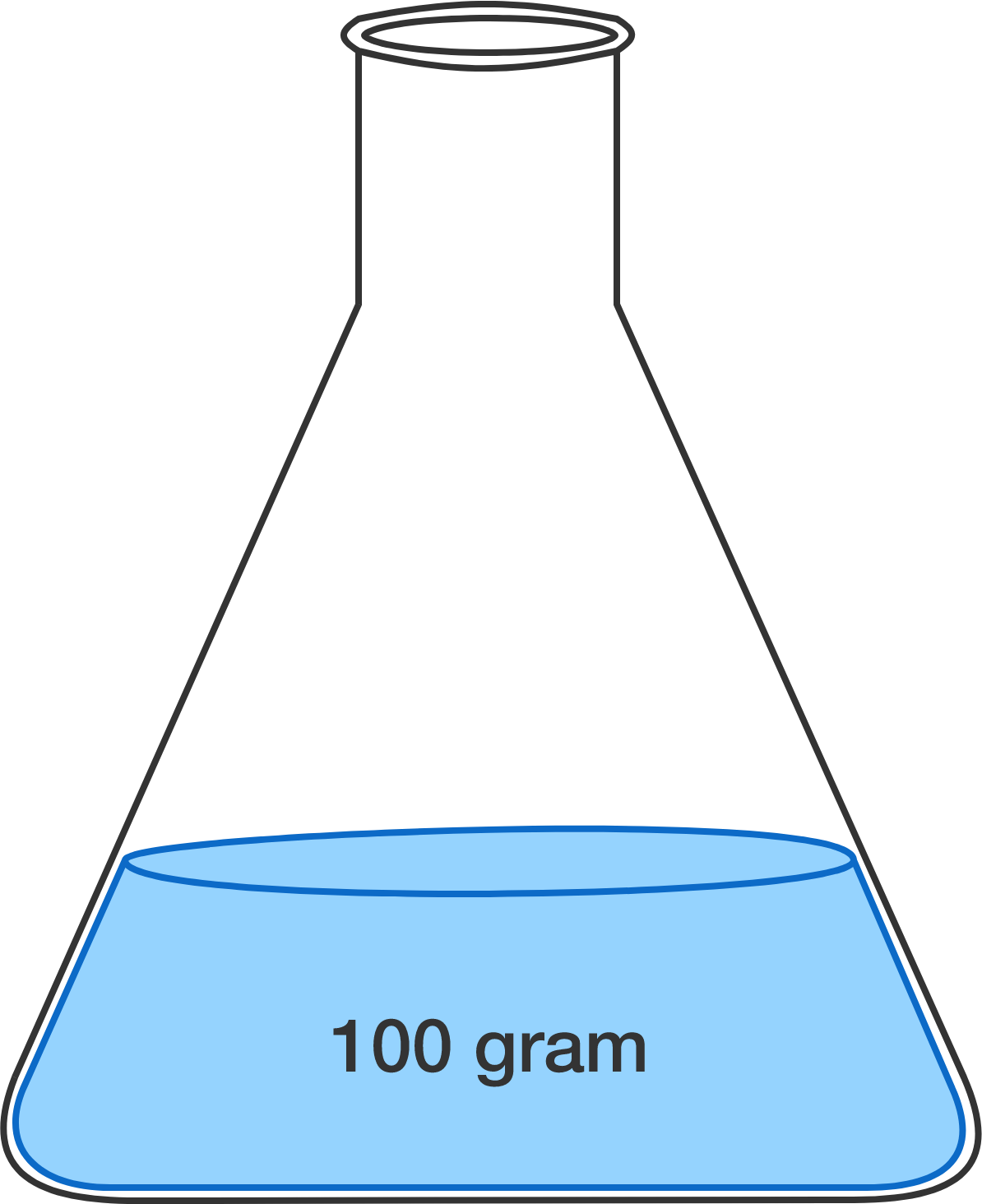# Water in a conical flaskA conical flask is filled with $\SI{100}{\gram}$ of water. How much force does the base of the flask exert on the water?

Take the acceleration due to gravity as $g = \SI[per-mode=symbol]{10}{\meter\per\second}^2.$ Neglect the atmospheric pressure.

×